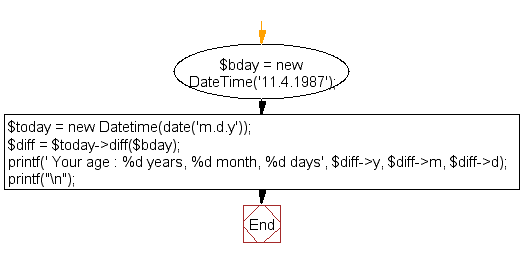﻿ PHP Date Exercise: Calculate the current age of a person - w3resource# PHP Date Exercises : Calculate the current age of a person

## PHP date: Exercise-18 with Solution

Write a PHP script to calculate the current age of a person.

Sample date of birth : 11.4.1987

Sample Solution:

PHP Code:

``````<?php
\$bday = new DateTime('11.4.1987'); // Your date of birth
\$today = new Datetime(date('m.d.y'));
\$diff = \$today->diff(\$bday);
printf(' Your age : %d years, %d month, %d days', \$diff->y, \$diff->m, \$diff->d);
printf("\n");
?>
```
```

Sample Output:

``` Your age : 30 years, 3 month, 0 days
```

N.B.: The result may varry for your system date and time.

Flowchart :PHP Code Editor:

Have another way to solve this solution? Contribute your code (and comments) through Disqus.

What is the difficulty level of this exercise?

﻿

## PHP: Tips of the Day

SQL injection that gets around mysql_real_escape_string()

Consider the following query:

```\$iId = mysql_real_escape_string("1 OR 1=1");
\$sSql = "SELECT * FROM table WHERE id = \$iId";
```

mysql_real_escape_string() will not protect you against this. The fact that you use single quotes (' ') around your variables inside your query is what protects you against this. The following is also an option:

```\$iId = (int)"1 OR 1=1";
\$sSql = "SELECT * FROM table WHERE id = \$iId";
```

Ref : https://bit.ly/32q3bJ7